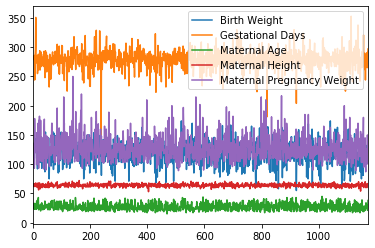## Lecture 9 – Data 100, Summer 2020¶

by Suraj Rampure

In :
import numpy as np
import pandas as pd
import matplotlib.pyplot as plt
import seaborn as sns

In :
births = pd.read_csv('baby.csv')

In :
births.head()

Out:
Birth Weight Gestational Days Maternal Age Maternal Height Maternal Pregnancy Weight Maternal Smoker
0 120 284 27 62 100 False
1 113 282 33 64 135 False
2 128 279 28 64 115 True
3 108 282 23 67 125 True
4 136 286 25 62 93 False
In :
births.shape

Out:
(1174, 6)

## Bar Plots¶

We often use bar plots to display distributions of a categorical variable:

In :
births['Maternal Smoker'].value_counts()

Out:
False    715
True     459
Name: Maternal Smoker, dtype: int64
In :
births['Maternal Smoker'].value_counts().plot(kind = 'bar');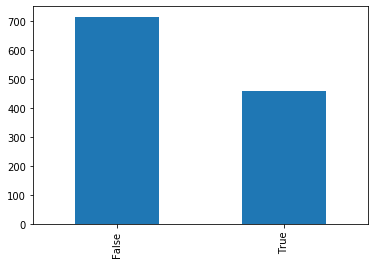Note: putting a semicolon after a plot call hides all of the unnecessary text that comes after it (the <matplotlib.axes_....>).

In :
sns.countplot(births['Maternal Smoker']);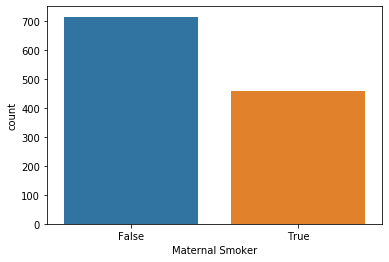But we can also use them to display a numerical variable that has been measured on individuals in different categories.

In :
# These are made up!
majors = ['Data Science', 'History', 'Biology', 'Business']
gpas = [3.35, 3.20, 2.98, 3.51]

In :
# What if we change bar to barh?
plt.bar(majors, gpas);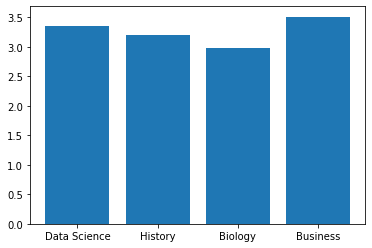In :
sns.barplot(majors, gpas);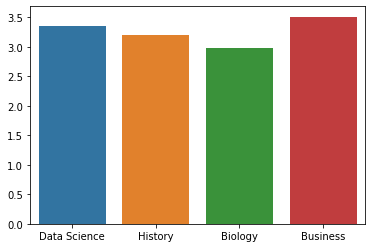## Rug plots¶

Used for visualizing a single quantitative variable. Rug plots show us each and every value.

In :
bweights = births["Birth Weight"]

In :
bweights

Out:
0       120
1       113
2       128
3       108
4       136
...
1169    113
1170    128
1171    130
1172    125
1173    117
Name: Birth Weight, Length: 1174, dtype: int64
In :
sns.rugplot(bweights);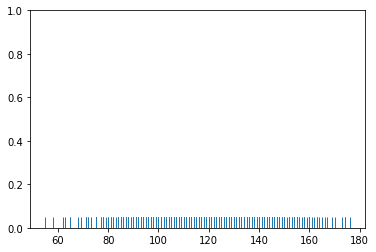## Histograms¶

Our old friend!

In :
# By default, you get some arbitrary bins. We usually like to pick our own.
plt.hist(bweights);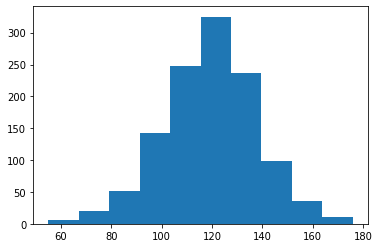In :
min(bweights), max(bweights)

Out:
(55, 176)
In :
bw_bins = range(50, 200, 5)

In :
plt.hist(bweights, bins=bw_bins, ec='w');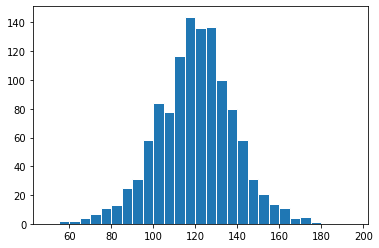In :
plt.hist(bweights, density=True, bins=bw_bins, ec='w');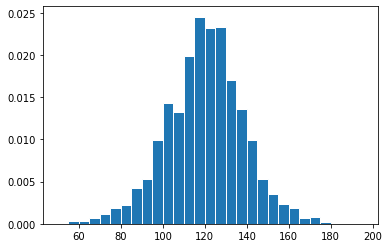In :
# alternative way of getting this plot
bweights.plot(kind = 'hist', density=True, bins=bw_bins, ec='w');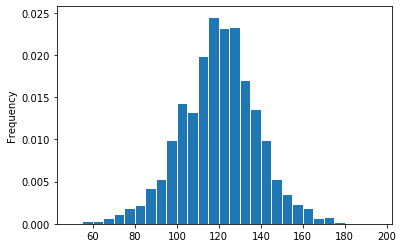Increasing bin width loses granularity, but this may be fine for our purposes.

In :
plt.hist(bweights, bins = np.arange(50, 200, 20), density=True, ec='w');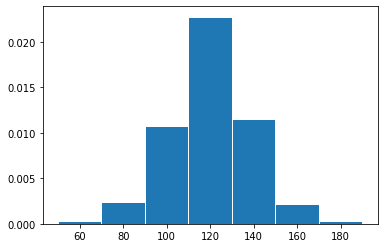The bin widths don't all need to be the same!

In :
plt.hist(bweights, bins = [50, 100, 120, 140, 200], density=True, ec='w');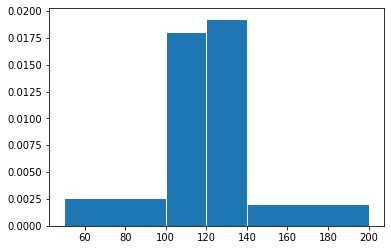## Density Curves¶

In :
sns.distplot(bweights);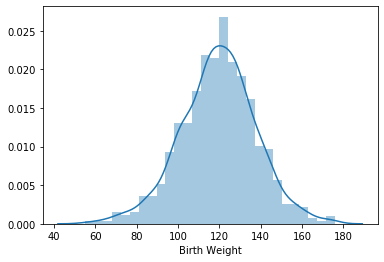Can isolate the histogram:

In :
sns.distplot(bweights, hist=False);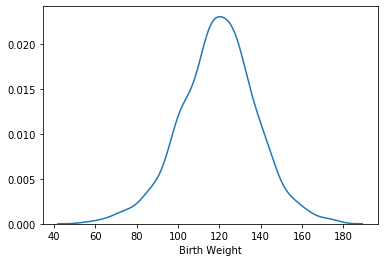Can even show a rugplot with it!

In :
sns.distplot(bweights, hist=False, rug=True);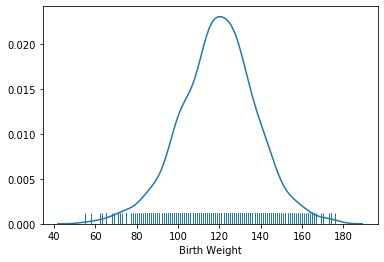## Box Plots¶

In :
plt.figure(figsize = (3, 6))
sns.boxplot(bweights, orient='v'); # the orient argument makes this vertical, to be consistent with the side by side ones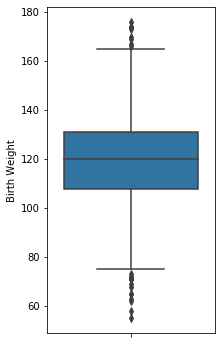In :
q1 = np.percentile(bweights, 25)
q2 = np.percentile(bweights, 50)
q3 = np.percentile(bweights, 75)
iqr = q3 - q1
whisk1 = q1 - 1.5*iqr
whisk2 = q3 + 1.5*iqr

whisk1, q1, q2, q3, whisk2

Out:
(73.5, 108.0, 120.0, 131.0, 165.5)

## Violin Plots¶

In :
plt.figure(figsize = (3, 6))
sns.violinplot(bweights, orient='v');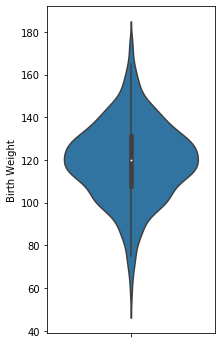## Overlaid Histograms and Density Curves¶

In :
sm_bweights = births[births['Maternal Smoker'] == True]['Birth Weight']
nsm_bweights = births[births['Maternal Smoker'] == False]['Birth Weight']

In :
sns.distplot(nsm_bweights, bins=bw_bins, hist_kws=dict(ec='w'), label='non smoker');
sns.distplot(sm_bweights, bins=bw_bins, hist_kws=dict(ec='w'), label='smoker');
plt.legend();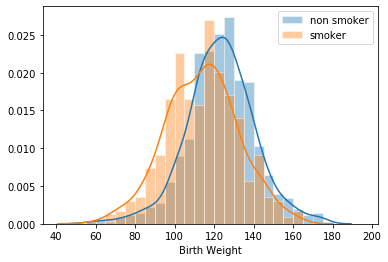In :
sns.distplot(nsm_bweights, bins=bw_bins, hist=False, hist_kws=dict(ec='w'), label='non smoker');
sns.distplot(sm_bweights, bins=bw_bins, hist=False, hist_kws=dict(ec='w'), label='smoker');
plt.legend();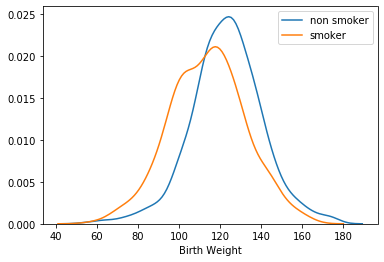In :
# Alpha adjusts transparency of the bins
plt.hist(nsm_bweights, bins = bw_bins, ec='w', density=True, alpha=0.4, label='non smoker');
plt.hist(sm_bweights, bins = bw_bins, ec='w', density=True, alpha=0.4, label='smoker');
plt.xlabel('Birth Weight')
plt.legend();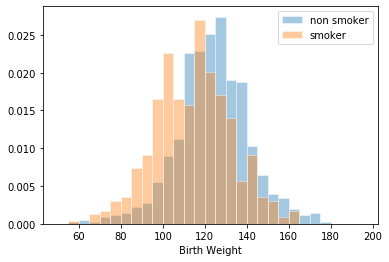## Side by side box plots and violin plots¶

In :
plt.figure(figsize=(5, 8))
sns.boxplot(data=births, x = 'Maternal Smoker', y = 'Birth Weight');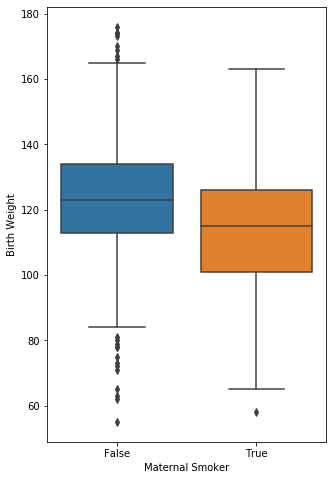In :
plt.figure(figsize=(5, 8))
sns.violinplot(data=births, x = 'Maternal Smoker', y = 'Birth Weight');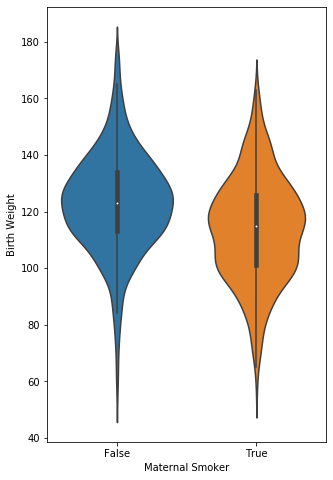A less fancy version of the above two plots:

In :
two_distributions = [nsm_bweights.values, sm_bweights.values]
groups = ['non-smokers', 'smokers']

In :
plt.boxplot(two_distributions, labels=groups);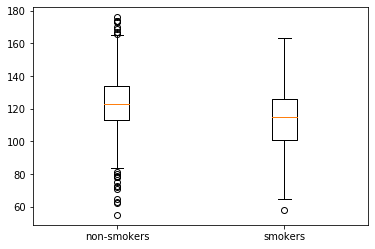In :
plt.violinplot(two_distributions);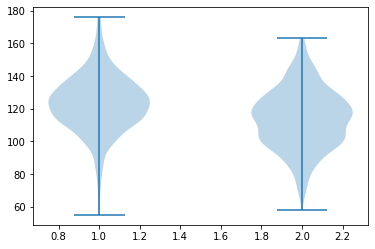## Scatter plots¶

In :
births

Out:
Birth Weight Gestational Days Maternal Age Maternal Height Maternal Pregnancy Weight Maternal Smoker
0 120 284 27 62 100 False
1 113 282 33 64 135 False
2 128 279 28 64 115 True
3 108 282 23 67 125 True
4 136 286 25 62 93 False
... ... ... ... ... ... ...
1169 113 275 27 60 100 False
1170 128 265 24 67 120 False
1171 130 291 30 65 150 True
1172 125 281 21 65 110 False
1173 117 297 38 65 129 False

1174 rows × 6 columns

In :
plt.scatter(births['Maternal Height'], births['Birth Weight'])
plt.xlabel('Maternal Height')
plt.ylabel('Birth Weight');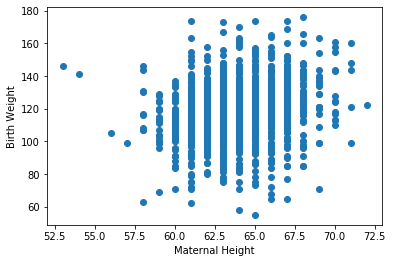In :
births['Birth Weight']

Out:
0       120
1       113
2       128
3       108
4       136
...
1169    113
1170    128
1171    130
1172    125
1173    117
Name: Birth Weight, Length: 1174, dtype: int64
In :
births['Maternal Height']

Out:
0       62
1       64
2       64
3       67
4       62
..
1169    60
1170    67
1171    65
1172    65
1173    65
Name: Maternal Height, Length: 1174, dtype: int64
In :
sns.scatterplot(data = births, x = 'Maternal Height', y = 'Birth Weight', hue = 'Maternal Smoker');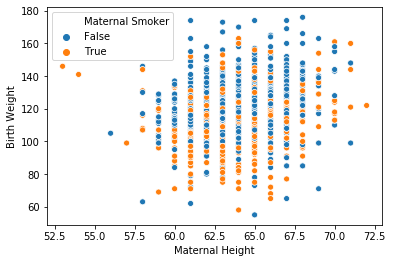In :
sns.lmplot(data = births, x = 'Maternal Height', y = 'Birth Weight', ci=False);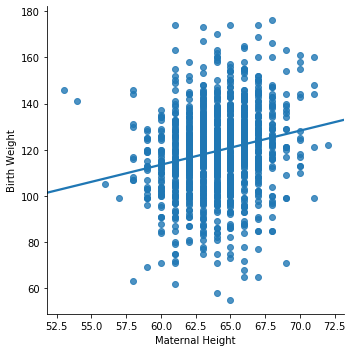In :
sns.jointplot(data = births, x = 'Maternal Height', y = 'Birth Weight');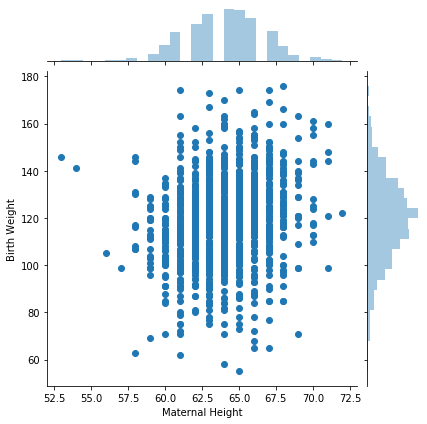## Hex plots and contour plots¶

In :
sns.jointplot(data = births, x = 'Maternal Height', y = 'Birth Weight', kind='hex');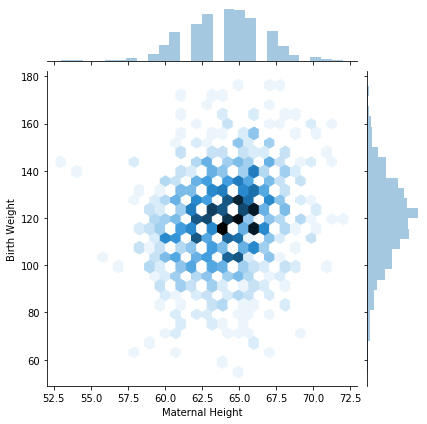In :
sns.jointplot(data = births, x = 'Maternal Height', y = 'Birth Weight', kind='kde');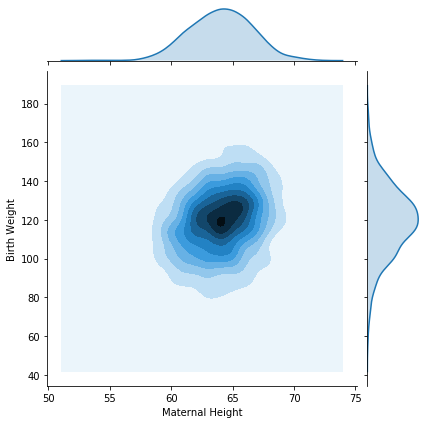## Bonus¶

Calling .plot() results in weird things!

In :
births.plot();